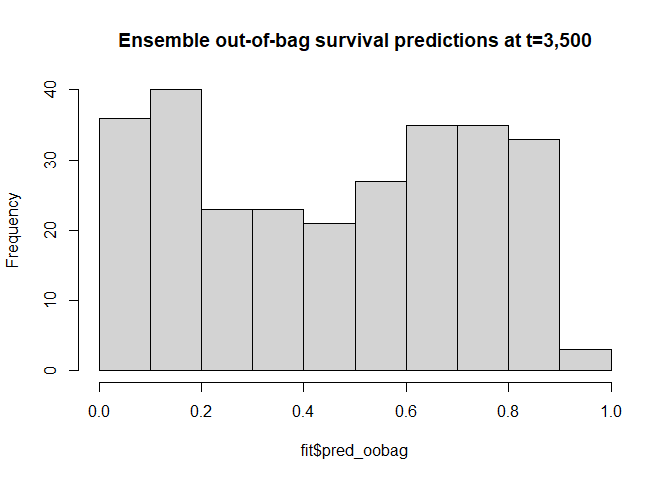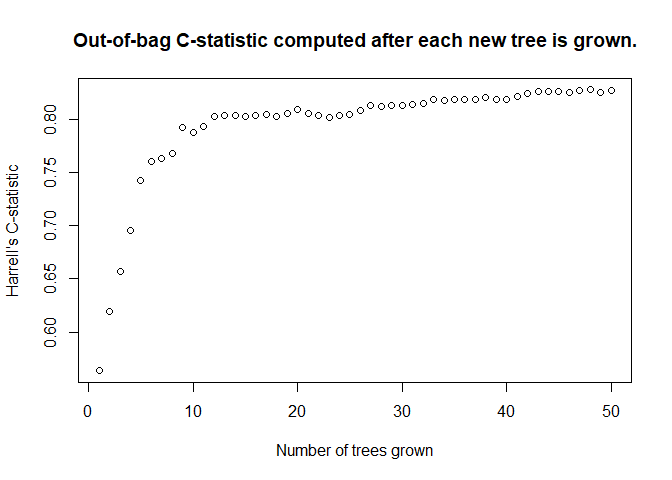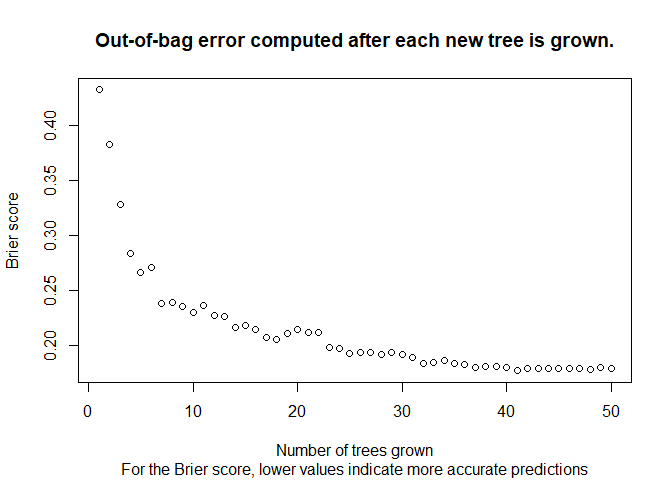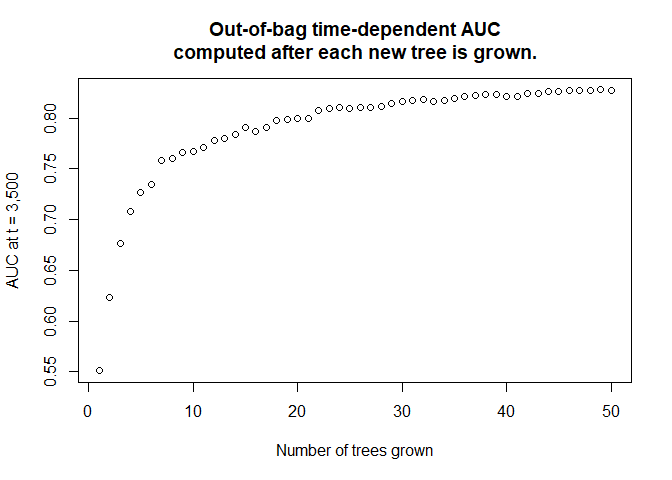# Out-of-bag predictions and evaluation


library(aorsf)
library(survival)
library(SurvMetrics)

## Out-of-bag data

In random forests, each tree is grown with a bootstrapped version of the training set. Because bootstrap samples are selected with replacement, each bootstrapped training set contains about two-thirds of instances in the original training set. The ‘out-of-bag’ data are instances that are not in the bootstrapped training set.

## Out-of-bag predictions and error

Each tree in the random forest can make predictions for its out-of-bag data, and the out-of-bag predictions can be aggregated to make an ensemble out-of-bag prediction. Since the out-of-bag data are not used to grow the tree, the accuracy of the ensemble out-of-bag predictions approximate the generalization error of the random forest. Out-of-bag prediction error plays a central role for some routines that estimate variable importance, e.g. negation importance.

Let’s fit an oblique random survival forest and plot the distribution of the ensemble out-of-bag predictions.


fit <- orsf(data = pbc_orsf,
formula = Surv(time, status) ~ . - id,
oobag_pred_type = 'surv',
oobag_pred_horizon = 3500)

hist(fit$pred_oobag, main = 'Ensemble out-of-bag survival predictions at t=3,500')Not surprisingly, all of the survival predictions are between 0 and 1. Next, let’s check the out-of-bag accuracy of fit:  # what function is used to evaluate out-of-bag predictions? fit$eval_oobag$stat_type #>  "Harrell's C-statistic" # what is the output from this function? fit$eval_oobag$stat_values #> [,1] #> [1,] 0.8410606 The out-of-bag estimate of Harrell’s C-statistic (the default method to evaluate out-of-bag predictions) is 0.8410606. ## Monitoring out-of-bag error As each out-of-bag data set contains about one-third of the training set, the out-of-bag error estimate usually converges to a stable value as more trees are added to the forest. If you want to monitor the convergence of out-of-bag error for your own oblique random survival forest, you can set oobag_eval_every to compute out-of-bag error at every oobag_eval_every tree. For example, let’s compute out-of-bag error after fitting each tree in a forest of 50 trees:  fit <- orsf(data = pbc_orsf, formula = Surv(time, status) ~ . - id, n_tree = 50, oobag_pred_horizon = 3500, oobag_eval_every = 1) plot( x = seq(1, 50, by = 1), y = fit$eval_oobag$stat_values, main = 'Out-of-bag C-statistic computed after each new tree is grown.', xlab = 'Number of trees grown', ylab = fit$eval_oobag$stat_type )In general, at least 500 trees are recommended for a random forest fit. We’re just using 50 in this case for better illustration of the out-of-bag error curve. Also, it helps to make run-times low whenever I need to re-compile the package vignettes. ## User-supplied out-of-bag evaluation functions In some cases, you may want more control over how out-of-bag error is estimated. For example, let’s use the Brier score from the SurvMetrics package:  oobag_fun_brier <- function(y_mat, s_vec){ # output is numeric vector of length 1 as.numeric( SurvMetrics::Brier( object = Surv(time = y_mat[, 1], event = y_mat[, 2]), pre_sp = s_vec, # t_star in Brier() should match oob_pred_horizon in orsf() t_star = 3500 ) ) } There are two ways to apply your own function to compute out-of-bag error. First, you can apply your function to the out-of-bag survival predictions that are stored in ‘aorsf’ objects, e.g:  oobag_fun_brier(y_mat = fit$data[, c('time', 'status')],
s_vec = fit$pred_oobag) #>  0.189913 Second, you can pass your function into orsf(), and it will be used in place of Harrell’s C-statistic:  fit <- orsf(data = pbc_orsf, formula = Surv(time, status) ~ . - id, n_tree = 50, oobag_pred_horizon = 3500, oobag_fun = oobag_fun_brier, oobag_eval_every = 1) plot( x = seq(1, 50, by = 1), y = fit$eval_oobag$stat_values, main = 'Out-of-bag error computed after each new tree is grown.', sub = 'For the Brier score, lower values indicate more accurate predictions', xlab = 'Number of trees grown', ylab = "Brier score" )We can also compute a time-dependent C-statistic instead of Harrell’s C-statistic (the default oob function):  oobag_fun_tdep_cstat <- function(y_mat, s_vec){ as.numeric( SurvMetrics::Cindex( object = Surv(time = y_mat[, 1], event = y_mat[, 2]), predicted = s_vec, t_star = 3500 ) ) } fit <- orsf(data = pbc_orsf, formula = Surv(time, status) ~ . - id, n_tree = 50, oobag_pred_horizon = 3500, oobag_fun = oobag_fun_tdep_cstat, oobag_eval_every = 1) plot( x = seq(50), y = fit$eval_oobag$stat_values, main = 'Out-of-bag time-dependent AUC\ncomputed after each new tree is grown.', xlab = 'Number of trees grown', ylab = "AUC at t = 3,500" )### Specific instructions on user-supplied functions User-supplied functions must: 1. have exactly two arguments named y_mat and s_vec. 2. return a numeric output of length 1 If either of these conditions is not true, an error will occur. A simple test to make sure your user-supplied function will work with the aorsf package is below:  # Helper code to make sure your oobag_fun function will work with aorsf # time and status values test_time <- seq(from = 1, to = 5, length.out = 100) test_status <- rep(c(0,1), each = 50) # y-matrix is presumed to contain time and status (with column names) y_mat <- cbind(time = test_time, status = test_status) # s_vec is presumed to be a vector of survival probabilities s_vec <- seq(0.9, 0.1, length.out = 100) # see 1 in the checklist above names(formals(oobag_fun_tdep_cstat)) == c("y_mat", "s_vec") #>  TRUE TRUE test_output <- oobag_fun_tdep_cstat(y_mat = y_mat, s_vec = s_vec) # test output should be numeric is.numeric(test_output) #>  TRUE # test_output should be a numeric value of length 1 length(test_output) == 1 #>  TRUE ## User-supplied functions for negation importance. Negation importance is based on the out-of-bag error, so of course you may be curious about what negation importance would be if it were computed using different statistics. The workflow for doing this is exactly the same as the example above, except we have to specify importance = 'negate' when we fit our model. Also, to speed up computations, I am not going to monitor out-of-bag error here.  fit_tdep_cstat <- orsf(data = pbc_orsf, formula = Surv(time, status) ~ . - id, n_tree = 500, oobag_pred_horizon = 3500, oobag_fun = oobag_fun_tdep_cstat, importance = 'negate') fit_tdep_cstat$importance
#>        bili         age      copper     protime     albumin       stage
#>  0.09223500  0.02119700  0.01914000  0.01500000  0.00937500  0.00750000
#>     ascites     spiders         ast         sex        chol      hepato
#>  0.00599000  0.00536500  0.00356800  0.00336000  0.00328200  0.00250000
#>       edema    platelet         trt    alk.phos        trig
#>  0.00144481  0.00114600 -0.00098900 -0.00234300 -0.00390600